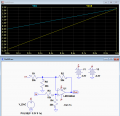# Using a unipolar DAC as a bipolar DAC

#### Abdel27

Joined Oct 10, 2019
4
Hello,

I'm currently working on a project that requires the use of an MCP475 DAC. Currently, it is being powered from the 3.3V source from on a Raspberry Pi and hence works in the range of 0V to 3.3V.

I need it to work in the range of -3.3V to +3.3V. By reading the spec sheets I found that I can use an Op-Amp circuit to achieve this. I have some experience with electronics but, obviously, not enough. I have attached the circuit diagram and the equations needed to calculate the required resistances.

My understanding is that V_DD = +3.3V. And when V_out = +3.3V, V_o = +3.3V. And again, when V_out = 0, V_o = - 3.3V. This gives you a pair of simultaneous equations which are then solved for the resistances. When I do the maths, I get the following:

R_2 = R_1
R_3 = 0
R_4 = R_4

So I thought maybe this means there is no R_3 and the values of R_2 and R_1 can be arbitrarily set as long as they're equal and then R_4 is also arbitrary. I build this circuit and nothing worked. What am I doing wrong? Please help me!

Thank you!

#### Papabravo

Joined Feb 24, 2006
20,143
In order for this circuit to work. you must allow Vin+ to be different from Vout. That is the purpose of the voltage divider formed by R3 and R4

#### Abdel27

Joined Oct 10, 2019
4
In order for this circuit to work. you must allow Vin+ to be different from Vout. That is the purpose of the voltage divider formed by R3 and R4
So the resistances R_3 = 0 and R_4 = R_4 cannot be correct, right? If so, what are the correct values?

#### Papabravo

Joined Feb 24, 2006
20,143
There is no unique solution. You have a system with more variables than you have equations. However if R3 = R4 then Vout is divided by 2 to create Vin+, and if R1=R2 the gain of the amplifier is 2. It is common for the gain of a non-inverting amplifier to be greater than 1. So what happens if you make all the resistors equal to say 4.75KΩ?

#### crutschow

Joined Mar 14, 2008
32,062
The calculation becomes much simpler for this particular case, if you add an extra resistor as shown below:
You need a gain of two for the DAC signal, which the differential amp below has [R2 = R4 = 2*R3, R3 = R1||R5 (Thevenin equivalent)].
The output needs to be offset by 3.3V which, with a differential gain of 2, is provided by the 1.65V from the voltage divider consisting of R1 and R5.

The LT spice simulation shows the output going from -3.3V to +3.3V for an input of 0V to +3.3V, as desired.

Note that the op amp must be a rail-rail type.#### Abdel27

Joined Oct 10, 2019
4
The calculation becomes much simpler for this particular case, if you add an extra resistor as shown below:
You need a gain of two for the DAC signal, which the differential amp below has [R2 = R4 = 2*R3, R3 = R1||R5 (Thevenin equivalent)].
The output needs to be offset by 3.3V which, with a differential gain of 2, is provided by the 1.65V from the voltage divider consisting of R1 and R5.

The LT spice simulation shows the output going from -3.3V to +3.3V for an input of 0V to +3.3V, as desired.

Note that the op amp must be a rail-rail type.

View attachment 190165
This is great! Thank you so much!

I just have a couple of quick questions. Will a UA741CP op-amp work? And what does the notation R1 || R5 mean?

#### crutschow

Joined Mar 14, 2008
32,062
Will a UA741CP op-amp work?
Not unless you have a plus and minus supply of at least 10V each for the 741.
Otherwise, as I stated, you need a rail-rail type op amp and the ancient 741 is definitely not.
what does the notation R1 || R5 mean?
It means R1 in parallel with R5.